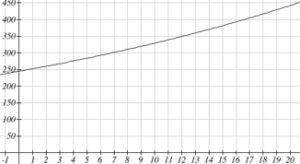## Solve Exponentials for Time: Logarithms

### Learning Outcomes

• Evaluate and rewrite logarithms using the properties of logarithms
• Use the properties of logarithms to solve exponential models for time
• Identify the carrying capacity in a logistic growth model
• Use a logistic growth model to predict growth

## Reversing an Exponent

Earlier, we found that since Olympia, WA had a population of 245 thousand in 2008 and had been growing at 3% per year, the population could be modeled by the equation

n = (1+0.03)n (245,000), or equivalently, P­n = 245,000(1.03)n.

Using this equation, we were able to predict the population in the future.

Suppose we wanted to know when the population of Olympia would reach 400 thousand. Since we are looking for the year n when the population will be 400 thousand, we would need to solve the equation

400,000 = 245,000(1.03)n

Dividing both sides by 245,000 gives

1.6327 = 1.03n

One approach to this problem would be to create a table of values, or to use technology to draw a graph to estimate the solution.From the graph, we can estimate that the solution will be around 16 to 17 years after 2008 (2024 to 2025). This is pretty good, but we’d really like to have an algebraic tool to answer this question. To do that, we need to introduce a new function that will undo exponentials, similar to how a square root undoes a square. For exponentials, the function we need is called a logarithm. It is the inverse of the exponential, meaning it undoes the exponential. While there is a whole family of logarithms with different bases, we will focus on the common log, which is based on the exponential 10x.

### Common Logarithm

The common logarithm, written log(x), undoes the exponential 10x

This means that log(10x) = x, and likewise 10log(x) = x

This also means the statement 10a = b is equivalent to the statement log(b) = a

log(x) is read as “log of x”, and means “the logarithm of the value x”. It is important to note that this is not multiplication – the log doesn’t mean anything by itself, just like √ doesn’t mean anything by itself; it has to be applied to a number.

### Example

Evaluate each of the following

1. log(100)
2. log(1000)
3. log(10000)
4. log(1/100)
5. log(1)

It is helpful to note that from the first three parts of the previous example that the number we’re taking the log of has to get 10 times bigger for the log to increase in value by 1.

Of course, most numbers cannot be written as a nice simple power of 10. For those numbers, we can evaluate the log using a scientific calculator with a log button.

### Example

Evaluate log(300)

With an equation, just like we can add a number to both sides, multiply both sides by a number, or square both sides, we can also take the logarithm of both sides of the equation and end up with an equivalent equation. This will allow us to solve some simple equations.

### Examples

1. Solve 10x = 1000
2. Solve 10x = 3
3. Solve 2(10x) = 8

This approach allows us to solve exponential equations with powers of 10, but what about problems like 2 = 1.03n from earlier, which have a base of 1.03? For that, we need the exponent property for logs.

### Properties of Logs: Exponent Property

$\log\left({{A}^{r}}\right)=r\log\left(A\right)$

To show why this is true, we offer a proof.

Since the logarithm and exponential undo each other, ${{10}^{\log{A}}}=A$.

So ${{A}^{r}}={{\left({{10}^{\log{A}}}\right)}^{r}}$

Utilizing the exponential rule that states ${{\left({{x}^{a}}\right)}^{b}}={{x}^{ab}}$,

${{A}^{r}}={{\left({{10}^{\log{A}}}\right)}^{r}}={{10}^{r\log{A}}}$

So then $\log\left({{A}^{r}}\right)=\log\left({{10}^{r\log{A}}}\right)$

Again utilizing the property that the log undoes the exponential on the right side yields the result

$\log\left({{A}^{r}}\right)=r\log{A}$

### Example

Rewrite log(25) using the exponent property for logs.

This property will finally allow us to answer our original question.

### Solving exponential equations with logarithms

1. Isolate the exponential. In other words, get it by itself on one side of the equation. This usually involves dividing by a number multiplying it.
2. Take the log of both sides of the equation.
3. Use the exponent property of logs to rewrite the exponential with the variable exponent multiplying the logarithm.
4. Divide as needed to solve for the variable.

### Example

If Olympia is growing according to the equation, P­n = 245(1.03)n, where n is years after 2008, and the population is measured in thousands. Find when the population will be 400 thousand.

A full walkthrough of this problem is available here.

### Try It

Alternatively, after applying the exponent property of logs on the right side, we could have evaluated the logarithms to decimal approximations and completed our calculations using those approximations, as you’ll see in the next example. While the final answer may come out slightly differently, as long as we keep enough significant values during calculation, our answer will be close enough for most purposes.

### Example

Polluted water is passed through a series of filters. Each filter removes 90% of the remaining impurities from the water. If you have 10 million particles of pollutant per gallon originally, how many filters would the water need to be passed through to reduce the pollutant to 500 particles per gallon?

More details about solving this scenario are available in this video.

### Try It

India had a population in 2008 of about 1.14 billion people. The population is growing by about 1.34% each year. If this trend continues, when will India’s population reach 1.2 billion?

## TIP

When you are solving growth problems, use the language in the question to determine whether you are solving for time, future value, present value or growth rate. Questions that uses words like “when”, “what year”, or “how long” are asking you to solve for time and you will need to use logarithms to solve them because the time variable in growth problems is in the exponent.